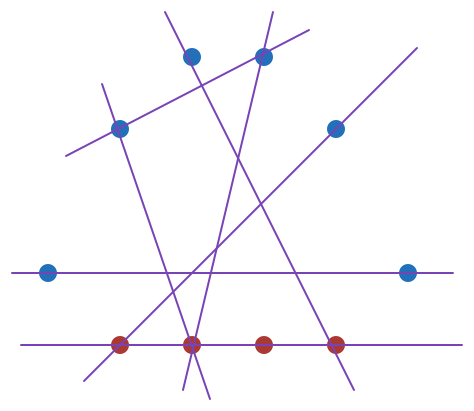Quantitative Finance

Combinations - Problem SolvingSuppose $10$ points are drawn on a plane such that exactly $4$ of the points are collinear and among the remaining points, no three points are collinear. How many distinct lines can be drawn by connecting any $2$ among these $10$ points?

How many ways can the integers $1,2,3,4,5,6$ be arranged such that $2$ is adjacent to either 1 or 3?

Details and assumptions

2 could be next to both 1 and 3.

Ten players were entered into a badminton tournament. The first round consisted of 5 matches, with each player in one match. How many different ways could the 10 players be matched against each other?

How many ways are there to pick two distinct numbers from $1$ to $11$ such that the sum of the two numbers is even?

The baseball teams SeaWolves and Raptors are playing for the championship. If there are $12$ players on the SeaWolves and $11$ players on Raptors, how many ways are there to choose $2$ players from the same team?

×